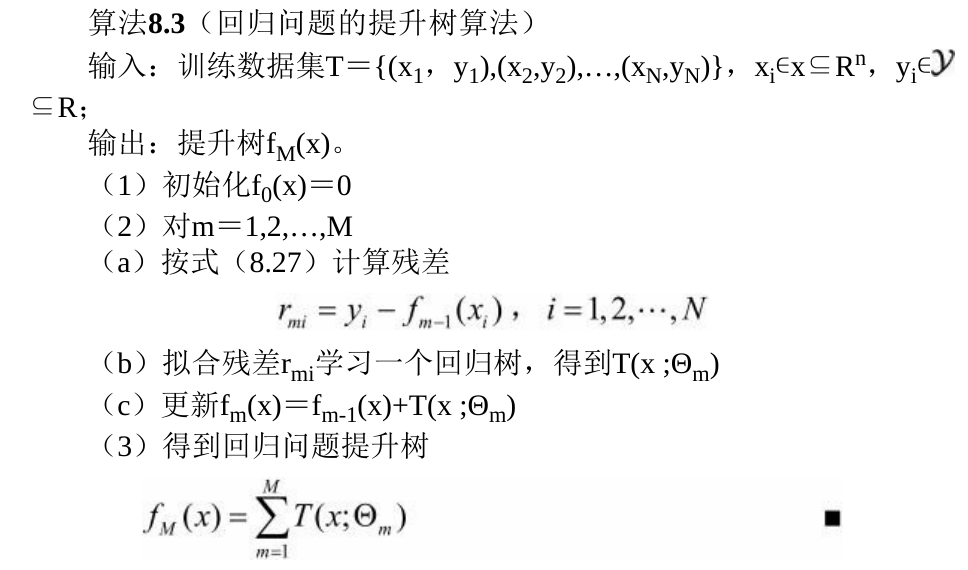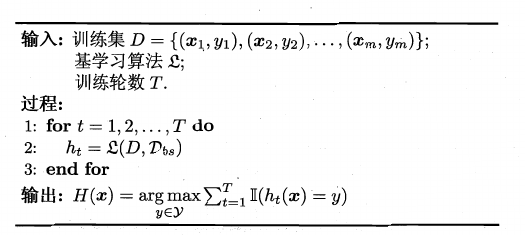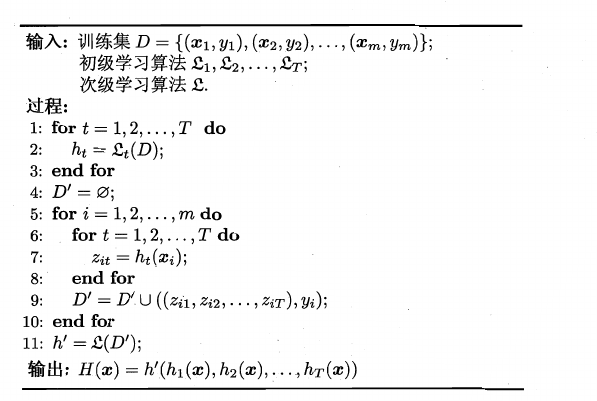# 集成学习

Posted by MaggicQ on April 28, 2019

# 集成学习

• 找到误差相互独立的基分类器
• 训练基分类器
• 合并基分类器的结果

## Boosting

Boosting方法训练基分类器时采用串行的方式，各个基分类器之间有依赖。它的基本思路是将基分类器层层叠加，每一层在训练的时候，对前一层基分类器分错的样本，给予更高的权重。测试时，根据各层分类器的结果的加权得到最终结果。

1. 初始化训练数据的权值分布 $D_{1}=\left(w_{11}, \cdots, w_{1 i}, \cdots, w_{1 N}\right), \quad w_{1 i}=\frac{1}{N}, \quad i=1,2, \cdots, N$

2. 对$M = 1,2, …m$

1. 使用具有权值分布$D_m$的训练数据集学习，得到基本分类器 $G_m(x) : \mathcal{X} \rightarrow \{-1, +1 \}$

2. 计算$G_m(x)$在训练数据集上的分类误差率 $e_{m}=P\left(G_{m}\left(x_{i}\right) \neq y_{i}\right)=\sum_{i=1}^{N} w_{m i} I\left(G_{m}\left(x_{i}\right) \neq y_{i}\right)$

3. 计算$G_m(x)$的系数$\alpha_{m}=\frac{1}{2} \log \frac{1-e_{m}}{e_{m}}$

4. 更新训练数据集的权值分布 $\begin{array}{c}{D_{m+1}=\left(w_{m+1,1}, \cdots, w_{m+1, i}, \cdots, w_{m+1, N}\right)} \\ {w_{m+1, i}=\frac{w_{m i}}{Z_{m}} \exp \left(-\alpha_{m} y_{i} G_{m}\left(x_{i}\right)\right), \quad i=1,2, \cdots, N}\end{array}$

这里$Z_m$是规范化因子$Z_{m}=\sum_{i=1}^{N} w_{m i} \exp \left(-\alpha_{m} y_{i} G_{m}\left(x_{i}\right)\right)$，它使$D_{m+1}$成为一个概率分布。

5. 构建基本分类器的线性组合$f(x)=\sum_{m=1}^{M} \alpha_{m} G_{m}(x)$ ，得到最终分类器：

• 不改变所给的训练数据，而不断改变训练数据权值的分布，使得训练数据在基本分类器的学习中起不同的作用。
• 利用基本分类器的线性组合来构建最终分类器。

### 梯度提升决策树GDBT的优点

• 预测阶段的计算速度快，树与树之间可并行化计算
• 在分布稠密的数据集上，泛化能力和表达能力都很好
• 采用决策树作为弱分类器使得GBDT模型具有较好的解释性和鲁棒性，能够自动发现特征间的高阶关系,并且也不需要对数据进行特殊的预处理如归一化等。

GDBT的局限性

• GBDT在高维稀疏的数据集上，表现不如支持向量机或者神经网络。
• GBDT在处理文本分类特征问题上，相对其他模型的优势不如它在处理数值特征时明显。
• 训练过程需要串行训练，只能在决策树内部采用一些局部并行的手段提高训练速度。

## Bagging

Bagging是并行式集成学习方法的最著名代表，它直接基于自主采样法（bootstrap sampling）。给定包含$m$个样本的数据集，我们先随机取出一个样本放入采样集中，再把该样本放回初始数据集，使得下次采样样本仍然可能被选中。照这样，我们可采样出$T$个含$m$个训练样本的采样集，然后基于每个采样集训练出一个基学习器，再将这些基学习器进行结合。这就是Bagging的基本流程。在对预测输出进行结合的时候，Bagging通常对分类任务采用简单投票法，对回归任务使用简单平均法。

Bagging的算法描述如下所示：## 结合策略

• 从统计的方面来看，学习任务的假设空间往往很大，可能有多个假设（模型）在训练集上达到同等性能，此时若使用单学习器可能因误选而导致泛化性能不佳，结合多个学习器则会减小这一风险。
• 从计算的方面来看，学习算法往往会陷入局部极小，通过多次运行之后进行结合，可降低陷入糟糕局部极小点的风险。
• 从表示的方面来看，某些学习任务的真实假设可能不在当前学习算法所考虑的假设空间里面，此时若使用单个学习器肯定无效，而通过结合多个学习器，由于相应的假设空间有所扩大，有可能学得更好的近似。

### 平均法

• 简单平均法
• 加权平均法（不同的集成学习方法可视为通过不同的方式来确定加权平均法中的基学习器的权重）

### 投票法

• 绝对多数投票法，即若某标记得票数过半，则预测该标记，否则拒绝预测。拒绝预测在可靠性较高的学习任务中是一个很好的机制。
• 相对多数投票法，即预测为得票数最多的标记，若同时有多个标记获得最高票，则从中随机选取一个。
• 加权投票法，与加权平均法类似。

### 学习法

Stacking先从初始数据集训练出出基学习器，然后“生成”一个新数据集用于训练次级学习器。在这个新数据集中，初级学习器的输出被当做样例输入特征，而初始样本的标记仍被当做样例标记。

Stacking的算法描述如下：## FAQ

### 常用的基分类器是什么？它有什么优点？

• 决策树可以较为方便地将样本的权重整合到训练过程中，而不需要使用过采样的方法来调整样本权重。

• 决策树的表达能力和泛化能力，可以通过调节树的层数来做折中。
• 数据样本的扰动对于决策树的影响较大，因此不同子样本集合生成的决策树基分类器随机性较大，这样的“不稳定学习器”更适合作为基分类器。此外，在决策树节点分裂的时候，随机地选择一个特征子集，从中找出最优分裂属性，很好地引入了随机性。

### Boosting和Bagging有何异同之处？

1. 训练方式不同。Boosting方法训练基分类器时采用串行的方式，各个基分类器之间有依赖。Bagging与Boosting的串行训练方式不同,Bagging方法在训练过程中，各基分类器之间无强依赖，可以进行并行训练。
2. 从消除基分类器的偏差和方差的角度来说，Boosting方法是通过逐步聚焦于基分类器分错的样本，减小集成分类器的偏差。Bagging方法则是采取分而治之的策略,通过对训练样本多次采样，并分别训练出多个不同模型，然后做综合，来减小集成分类器的方差。

### 什么是模型的偏差和方差，Boosting和Bagging方法与偏差和方差的关系是什么？

Bagging能够提高弱分类器性能的原因是降低了方差，Boosting能够提升弱分类器性能的原因是降低了偏差。从模型的角度来理解这个解释：

Bagging对n个独立不相关的模型的预测结果取平均，方差是原来单个模型的1/n。（假设有$n$个两两独立的随机变量，方差为$\sigma^2$，其平均值$\frac{\sum X_i}{n}$的方差为$\frac{\sigma^2}{n}$ ）。当然，模型之间不可能完全独立。为了追求模型的独立性，诸多Bagging的方法做了不同的改进。比如在随机森林算法中，每次选取节点分裂属性时，会随机抽取一个属性子集，而不是从所有属性中选取最优属性，这就是为了避免弱分类器之间过强的相关性。通过训练集的重采样也能够带来弱分类器之间的一定独立性，从而降低Bagging后模型的方差。

### XGBoost、LightGBM与GBDT的联系和区别有哪些？

XGBoost vs GBDT

• GBDT是机器学习算法，XGBoost是该算法的工程实现。

• 在使用决策树作为基分类器时，XGBoost显式地加入了正则项来控制模型的复杂度，有利于防止过拟合，从而提高模型的泛化能力。

• 传统的GBDT采用决策树作为基分类器，XGBoost支持多种类型的基分类器，比如线性分类器。

• 传统的GBDT在每轮迭代时使用全部的数据，XGBoost则采用了与随机森林相似的策略，支持对数据进行采样。

• 传统的GBDT没有设计对缺失值进行处理，XGBoost能够自动学习出缺失值的处理策略。

• GBDT在模型训练时只使用了代价函数的一阶导数信息，XGBoost对代价函数进行二阶泰勒展开，可以同时使用一阶和二阶导数。

lightGBM vs XGBoost

• xgboost采用的是level-wise的分裂策略，而lightGBM采用了leaf-wise的策略，区别是xgboost对每一层所有节点做无差别分裂，可能有些节点的增益非常小，对结果影响不大，但是xgboost也进行了分裂，带来了务必要的开销。 leaft-wise的做法是在当前所有叶子节点中选择分裂收益最大的节点进行分裂，如此递归进行，很明显leaf-wise这种做法容易过拟合，因为容易陷入比较高的深度中，因此需要对最大深度做限制，从而避免过拟合。

• lightgbm使用了基于histogram的决策树算法，这一点不同与xgboost中的 exact 算法，histogram算法在内存和计算代价上都有不小优势。

• lightgbm支持直接输入categorical 的feature

xgboost使用经验总结

• 多类别分类时，类别需要从0开始编码
• Watchlist不会影响模型训练。
• 类别特征必须编码，因为xgboost把特征默认都当成数值型的
• 调参：Notes on Parameter Tuning 以及 Complete Guide to Parameter Tuning in XGBoost (with codes in Python)
• 训练的时候，为了结果可复现，记得设置随机数种子。
• XGBoost的特征重要性是如何得到的？某个特征的重要性（feature score），等于它被选中为树节点分裂特征的次数的和，比如特征A在第一次迭代中（即第一棵树）被选中了1次去分裂树节点，在第二次迭代被选中2次…..那么最终特征A的feature score就是 1+2+….

## 参考

lightgbm,xgboost,gbdt的区别与联系

XGBoost浅入浅出

XGBoost: A Scalable Tree Boosting System